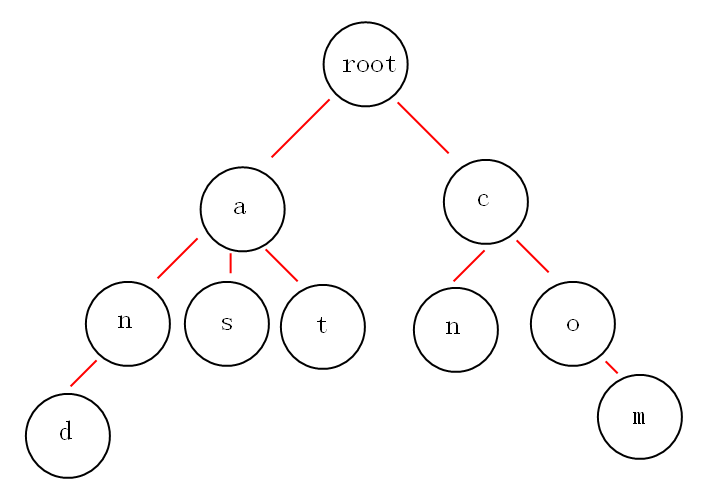# 概念

Trie树的名字有很多，比如字典树，前缀树等等。第一：根节点不包含字符，除根节点外的每一个子节点都包含一个字符。
第二：从根节点到某一节点，路径上经过的字符连接起来，就是该节点对应的字符串。
第三：每个单词的公共前缀作为一个字符节点保存。


（1）词频统计：

（2）前缀匹配：

# 实现

## 1. 数据模型

/**

*/
type TrieNode struct {
/* 子节点 */
childs []*TrieNode

freq int

nodeChar byte
}


## 2. 添加字符串

func addNode(root *TrieNode, word string) {
if len(word) == 0 {
return
}
//找到word的第一个字符在root节点的第k个子节点
k := word - 'a'

if root.childs[k] == nil {
root.childs[k] = &TrieNode{
freq:     1,
nodeChar: word,
childs:   make([]*TrieNode, 26),
}
}
nextWord := word[1:]
if len(nextWord) == 0 {
root.childs[k].freq++
return
}
addNode(root.childs[k], nextWord)
}


## 3. 删除操作

func deleteNode(root *TrieNode, word string) {
if len(word) == 0 {
return
}
//找到word的第一个字符在root节点的第k个子节点
k := word - 'a'
if root.childs[k] == nil {
return
}
nextWord := word[1:]
if len(nextWord) == 0 && root.childs[k].freq > 0 {
root.childs[k].freq--
}
deleteNode(root.childs[k], nextWord)
}


## 4. 获取单词频率

/**

*/
func get(root *TrieNode, word string) int {
if len(word) == 0 {
return 0
}
k := word - 'a'
if root.childs[k] == nil {
return 0
}
nextWord := word[1:]
if len(nextWord) == 0 {
return root.childs[k].freq
}
return get(root.childs[k], nextWord)
}


## 5. 测试

func GetRandomString(l int) string {
str := "abcdefghijklmnopqrstuvwxyz"
bytes := []byte(str)
result := []byte{}
r := rand.New(rand.NewSource(time.Now().UnixNano()))
for i := 0; i < l; i++ {
result = append(result, bytes[r.Intn(len(bytes))])
}
return string(result)
}

//----------------------------

package trie_tree

import (
"fmt"
"testing"
"time"
)

func prepareData() *TrieNode {
root := &TrieNode{
freq:     -1,
nodeChar: 0,
childs:   make([]*TrieNode, 26),
}
for i := 0; i < 1000000; i++ {
addNode(root, GetRandomString(32))
}
return root
}

func TestTrie(t *testing.T) {
root := prepareData()
str := GetRandomString(32)
addNode(root, str)
fmt.Println(str)
start := time.Now().UnixNano()
freq := get(root, str) - 1
end := time.Now().UnixNano()
fmt.Println(freq)
fmt.Println(end - start)
}